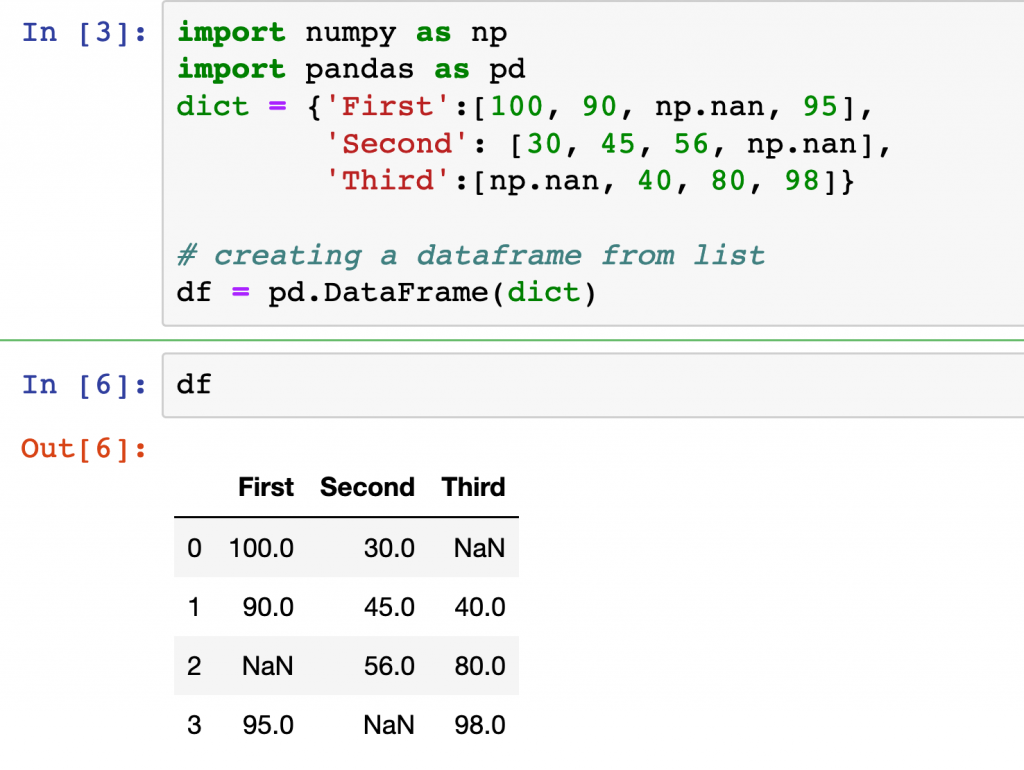# A Guide To KNN Imputation For Handling Missing Values

How to handle missing data in your dataset with Scikit-Learn’s KNN Imputer

Missing Values in the dataset is one heck of a problem before we could get into Modelling. A lot of machine learning algorithms demand those missing values to be imputed before proceeding further.

# What are Missing Values?

A missing value can be defined as the data value that is not captured nor stored for a variable in the observation of interest. There are 3 types of missing values –

Missing Completely at Random (MCAR)

MCAR occurs when the missing on the variable is completely unsystematic. When our dataset is missing values completely at random, the probability of missing data is unrelated to any other variable and unrelated to the variable with missing values itself. For example, MCAR would occur when data is missing because the responses to a research survey about depression are lost in the mail.

Missing at Random (MAR)

MAR occurs when the probability of the missing data on a variable is related to some other measured variable but unrelated to the variable with missing values itself. For example, the data values are missing because males are less likely to respond to a depression survey. In this case, the missing data is related to the gender of the respondents. However, the missing data is not related to the level of depression itself.

Missing Not at Random (MNAR)

MNAR occurs when the missing values on a variable are related to the variable with the missing values itself. In this case, the data values are missing because the respondents failed to fill in the survey due to their level of depression.

# Effects of Missing Values

Having missing values in our datasets can have various detrimental effects. Here are a few examples –

1. Missing data can limit our ability to perform important data science tasks such as converting data types or visualizing data
2. Missing data can reduce the statistical power of our models which in turn increases the probability of Type II error. Type II error is the failure to reject a false null hypothesis.
3. Missing data can reduce the representativeness of the samples in the dataset.
4. Missing data can distort the validity of the scientific trials and can lead to invalid conclusions.

# KNN Imputer

The popular (computationally least expensive) way that a lot of Data scientists try is to use mean/median/mode or if it’s a Time Series, then lead or lag record.

There must be a better way — that’s also easier to do — which is what the widely preferred KNN-based Missing Value Imputation.

scikit-learn‘s v0.22 natively supports KNN Imputer — which is now officially the easiest + best (computationally least expensive) way of Imputing Missing Value. It’s a 3-step process to impute/fill NaN (Missing Values). This post is a very short tutorial of explaining how to impute missing values using KNNImputer

Make sure you update your scikit-learn

`pip3 install -U scikit-learn`

`from sklearn.impute import KNNImputer`

# How does it work?

According scikit-learn docs:

Each sample’s missing values are imputed using the mean value from n_neighbors nearest neighbors found in the training set. Two samples are close if the features that neither is missing are close. By default, a euclidean distance metric that supports missing values, nan_euclidean_distances, is used to find the nearest neighbors.

## Creating Dataframe with Missing Values

`import numpy as npimport pandas as pddict = {‘First’:[100, 90, np.nan, 95],  ‘Second’: [30, 45, 56, np.nan],  ‘Third’:[np.nan, 40, 80, 98]}  # creating a dataframe from list df = pd.DataFrame(dict)`At this point, You’ve got the dataframe df with missing values.

# 2. Initialize KNNImputer

You can define your own n_neighbors value (as its typical of KNN algorithm).

`imputer = KNNImputer(n_neighbors=2)`

# 3. Impute/Fill Missing Values

`df_filled = imputer.fit_transform(df)`

## Conclusion

As you can see above, that’s the entire missing value imputation process is. It’s as simple as just using mean or median but more effective and accurate than using a simple average. Thanks to the new native support in scikit-learn, This imputation fit well in our pre-processing pipeline.